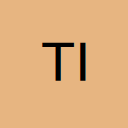# Using conditions to replace the value of a cell just modified - VBA

• Hi, so what I've been trying to do without success is to make excell automatically replace the content of a cell if it's in column "B" and its value has just been changed to "Distribute".
Then, if the content of the cell in the same row but in column E is empty the value to replace "Distribute" will be copied from cell M22 in another worksheet.
Or, If the content of the cell in the same row but in column E is NOT empty the value to replace "Distribute" will be copied from cell M24 in another worksheet.

How should I proceed to make it happen?

Ps: I did'nt get to the part where I would copy different values after checking the column E.
The code I was trying is the following:

[VBA]
Private Sub Worksheet_Change2(ByVal Target As Range)
Dim s1 As Worksheet, s2 As Worksheet, thiscolumn As Long, thisrow As Long
Set s1 = Sheets("Control")
Set s2 = Sheets("Graph")
If Not Intersect(Target, Range("B:B")) Is Nothing Then
thisrow = Target.Row
thiscolumn = Target.Column
If InStr(1, Range(Cells(thisrow, thiscolumn)), "Distribute") Then
s2.Range(Cells(20, 13)).Copy
s1.Range(Cells(thisrow, thiscolumn)).PasteSpecial x1PasteValues
End If
End If
End Sub
[/VBA]

• Hello,

Have you tested your code with : Private Sub Worksheet_Change(ByVal Target As Range)

i.e. without the number 2 ...

By the way, you cannot have Worksheet_Change Events within the same sheet ...

which means that you do need to merge both codes into a single one ...

Hope this will help

If you feel like saying "Thank You" for the help received, do not hesitate to click the "Thumbs Up" icon, below, in the bottom right corner• Thank you for the help Carim. I wasn't aware of that.
I merged it with the other code and did a few changes to it, since it still wasn't working.

now I have the following code:

[VBA]Private Sub Worksheet_Change(ByVal Target As Range)
Dim s1 As Worksheet, s2 As Worksheet, lr As Long, s3 As Worksheet, thiscolumn As Long
Set s1 = Sheets("Controle")
Set s2 = Sheets("Distribuição")
Set s3 = Sheets("Gráfico")
Dim thisrow As Long
If Target.Column = 6 Then
thisrow = Target.Row
If Target.Value = "Atribuído" Then
lr = s2.Range("A" & Rows.Count).End(xlUp).Row
s1.Range(Cells(thisrow, 1), Cells(thisrow, 2)).Copy s2.Range("A" & lr + 1)
s2.Range("C" & lr + 1) = Date
s2.Range("A:B").ClearFormats
End If
End If
If Not Intersect(Target, Range("B:B")) Is Nothing Then
thiscolumn = Target.Column
If Target.Value = "Distribuir" Then
s3.Range(Cells(20, 13)).Copy s1.Range(Cells(thisrow, thiscolumn)
End If
End If
End Sub[/VBA]

The first part is working fine (the code I already had), but in the second part I'm getting an error when trying to copy the values in
[VBA]s3.Range(Cells(20, 13)).Copy s1.Range(Cells(thisrow, thiscolumn)[/VBA]

Ps: I changed the names of the values and worksheets to portuguese to avoid mistakes when importing in the original file

• I've managed to make it work, probably not the best written code, but it works.
Thank you for the help.

Working Code:

[VBA]Private Sub Worksheet_Change(ByVal Target As Range)
Dim thisrow As Long
If Not Intersect(Target, Range("B:B")) Is Nothing Then
If Target.Value = "Distribuir" Then
thisrow = Target.Row
If Cells(thisrow, 5).Value <> "" Then
Worksheets("Gráfico").Range("M22").Copy
Else
Worksheets("Gráfico").Range("M20").Copy
End If
ActiveCell.PasteSpecial xlPasteValues
End If
End If
End Sub[/VBA]

If you feel like saying "Thank You" for the help received, do not hesitate to click the "Thumbs Up" icon, below, in the bottom right corner